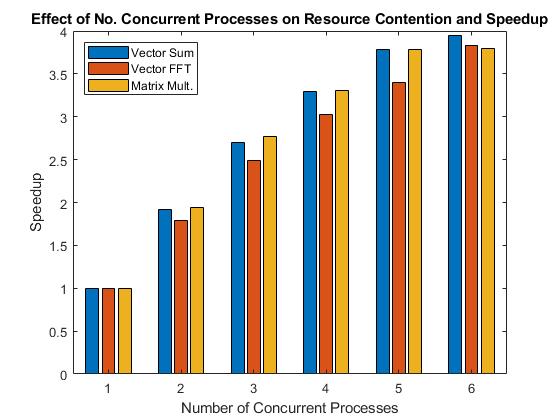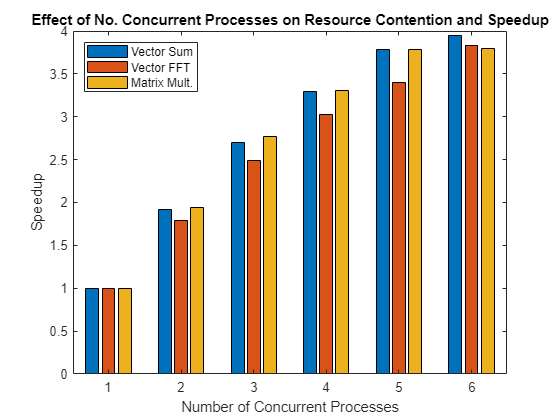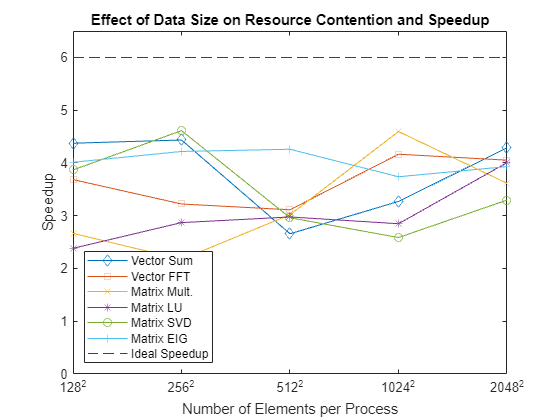# Resource Contention in Task Parallel Problems

This example shows why it is difficult to give a concrete answer to the question "How will my parallel code perform on my multicore machine or on my cluster?"

The answer most commonly given is "It depends on your code as well as your hardware" and this example will try to explain why this is all one can say without more information.

This example uses parallel code running on workers on the same multicore CPU and highlights the problem of contention for memory access. To simplify the problem, this example benchmarks your computer's ability to execute task parallel problems that do not involve disk IO. This allows you to ignore several factors that might affect parallel code execution, such as:

• Amount of inter-process communication

• Network bandwidth and latency for inter-process communication

• Process startup and shutdown times

• Time to dispatch requests to the processes

• Disk IO performance

This leaves only:

• Time spent executing task parallel code

This figure shows the speedup achieved when performing operations in parallel on a number of concurrent processes.### Analogy for Resource Contention and Efficiency

To understand why it is worthwhile to perform such a simple benchmark, consider the following example: If one person can fill one bucket of water, carry it some distance, empty it, and take it back to refill it in one minute, how long will it take two people to go the same round trip with one bucket each? This simple analogy closely reflects the task parallel benchmarks in this example. At first glance, it seems absurd that there should be any decrease in efficiency when two people are simultaneously doing the same thing as compared to one person.

### Competing for One Pipe: Resource Contention

If all things are perfect in our previous example, two people complete one loop with a bucket of water each in one minute. Each person quickly fills one bucket, carries the bucket over to the destination, empties it and walks back, and they make sure never to interfere or interrupt one another.

However, imagine that they have to fill the buckets from a single, small water hose. If they arrive at the hose at the same time, one would have to wait. This is one example of a contention for a shared resource. Maybe the two people don't need to simultaneously use the hose, and the hose therefore serves their needs; but if you have 10 people transporting a bucket each, some might always have to wait.

In the analogy, the water hose corresponds to the computer hardware, in particular memory. If multiple programs are running simultaneously on one CPU core each, and they all need access to data that is stored in the computer's memory, some of the programs may have to wait because of limited memory bandwidth.

### Same Hose, Different Distance, Different Results

Imagine that there is contention at the hose when two people are carrying one bucket each, but then the task is changed and the people must carry the water quite a bit further away from the hose. When performing this modified task, the two people spend a larger proportion of their time doing work, i.e., walking with the buckets, and a smaller proportion of their time contending over the shared resource, the hose. They are therefore less likely to need the hose at the same time, so this modified task has a higher parallel efficiency than the original one.

In the case of the benchmarks in this example, this corresponds on the one hand to running programs that require lots of access to the computer's memory, but they perform very little work with the data once fetched. If, on the other hand, the programs perform lots of computations with the data, it becomes irrelevant how long it took to fetch the data, the computation time will overshadow the time spent waiting for access to the memory.

The predictability of the memory access of an algorithm also effects how contended the memory access will be. If the memory is accessed in a regular, predictable manner, there will be less contention than if the memory is accessed in an irregular manner. This can be seen further below, where, for example, singular value decomposition calculations result in more contention than matrix multiplication.

### Start a Parallel Pool

Close any existing parallel pools and start a process-based parallel pool using `parpool`. With default preferences, `parpool` starts a pool on the local machine with one worker per physical CPU core, up to the preferred number of workers.

```delete(gcp("nocreate")); % Close any existing parallel pools p = parpool("Processes");```
```Starting parallel pool (parpool) using the 'Processes' profile ... Connected to the parallel pool (number of workers: 6). ```
`poolSize = p.NumWorkers;`

### The Timing Function

A timing function, `timingFcn`, is provided at the end of this example. The timing function executes a function five times within an `spmd` statement, and retains the minimum execution time observed for a given level of concurrency.

As stated above, this example benchmarks task parallel problems, measuring only the actual runtime. This means that the example does not benchmark the performance of MATLAB®, Parallel Computing Toolbox™, or the `spmd` language construct. Instead, the example benchmarks the ability of the OS and hardware to simultaneously run multiple copies of a program.

### Set up Benchmarking Problem

Create an input matrix large enough that it needs to be brought from the computer's memory onto the CPU each time it is processed. That is, make it large enough to cause resource contention.

```sz = 2048; m = rand(sz*sz,1);```

#### Summation Operations

A function for performing repeated summations on a single array, `sumOp`, is provided at the end of this example. Since the summation operations are computationally lightweight, you can expect to see resource contention when running multiple copies of this function simultaneously with a large input array. Consequently, you should expect it to take longer to evaluate the summation function when performing multiple such evaluations concurrently than it takes to execute a single such evaluation on an otherwise idle CPU.

#### Fast Fourier Transform Operations

A function for performing repeated Fast Fourier Transforms (FFTs) on a vector, `fftOp`, is provided at the end of this example. FFT operations are more computationally intensive than summation operations, and therefore you should expect not to see the same performance degradations when concurrently evaluating multiple calls to the FFT function as with calls to the summation function.

#### Matrix Multiplication Operations

A function for performing matrix multiplication, `multOp`, is provided at the end of this example. The memory access in matrix multiplication is very regular and so this operation therefore has the potential to be executed quite efficiently in parallel on a multicore machine.

### Investigate the Resource Contention

Measure how long it takes to simultaneously evaluate N summation functions on N workers for values of N from 1 to the size of the parallel pool.

`tsum = timingFcn(@() sumOp(m), 1:poolSize);`
```Execution times: 0.279930, 0.292188, 0.311675, 0.339938, 0.370064, 0.425983 ```

Measure how long it takes to simultaneously evaluate N FFT functions on N workers for values of N from 1 to the size of the parallel pool.

`tfft = timingFcn(@() fftOp(m),1:poolSize);`
```Execution times: 0.818498, 0.915932, 0.987967, 1.083160, 1.205024, 1.280371 ```

Measure how long it takes to simultaneously evaluate N matrix multiplication functions on N workers for values of N from 1 to the size of the parallel pool.

```m = reshape(m,sz,sz); tmtimes = timingFcn(@() multOp(m),1:poolSize);```
```Execution times: 0.219166, 0.225956, 0.237215, 0.264970, 0.289410, 0.346732 ```
`clear m`

Combine the timing results into a single array and calculate the speedup achieved by running multiple function invocations concurrently.

```allTimes = [tsum(:), tfft(:), tmtimes(:)]; speedup = (allTimes(1,:)./allTimes).*((1:poolSize)');```

Plot the results in a bar chart. This chart shows the speedup with what is known as weak scaling. Weak scaling is where the number of processes/processors varies, and the problem size on each process/processor is fixed. This has the effect of increasing the total problem size as you increase the number of processes/processors. On the other hand, strong scaling is where the problem size is fixed and the number of processes/processors varies. The effect of this is that as you increase the number of processes/processors, the work done by each process/processor decreases.

```bar(speedup) legend('Vector Sum', 'Vector FFT', 'Matrix Mult.', ... 'Location', 'NorthWest') xlabel('Number of Concurrent Processes'); ylabel('Speedup') title(['Effect of No. Concurrent Processes on ', ... 'Resource Contention and Speedup']);```### Effect on Real Applications

Looking at the graph above, you can see that problems can scale differently on the same computer. Considering the fact that other problems and computers may show very different behavior, it should become clear why it is impossible to give a general answer to the question "How will my (parallel) application perform on my multi-core machine or on my cluster?" The answer to that question truly depends on the application and the hardware in question.

### Measure Effect of Data Size on Resource Contention

Resource contention does not depend only on the function being executed, but also on the size of the data being processed. To illustrate this, you will measure the execution times of various functions with various sizes of input data. As before, you are benchmarking the ability of our hardware to perform these computations concurrently, and not MATLAB or its algorithms. More functions will be considered than before so that you can investigate the effects of different memory access patterns as well as the effects of different data sizes. As well as the functions described above, you will also benchmark using

Define the data sizes specify the operations that well be used in the tests.

```szs = [128, 256, 512, 1024, 2048]; operations = {'Vector Sum', 'Vector FFT', 'Matrix Mult.', 'Matrix LU', ... 'Matrix SVD', 'Matrix EIG'};```

Loop through the different data sizes and the functions, and measure the sequential execution time and the time it takes to execute concurrently on all of the workers in the parallel pool.

```speedup = zeros(length(szs), length(operations)); % Loop over the data sizes for i = 1:length(szs) sz = szs(i); fprintf('Using matrices of size %d-by-%d.\n', sz, sz); j = 1; % Loop over the different operations for f = [{@sumOp; sz^2; 1}, {@fftOp; sz^2; 1}, {@multOp; sz; sz}, ... {@lu; sz; sz}, {@svd; sz; sz}, {@eig; sz; sz}] op = f{1}; nrows = f{2}; ncols = f{3}; m = rand(nrows, ncols); % Compare sequential execution to execution on all workers tcurr = timingFcn(@() op(m), [1, poolSize]); speedup(i, j) = tcurr(1)/tcurr(2)*poolSize; j = j + 1; end end```
```Using matrices of size 128-by-128. ```
```Execution times: 0.000496, 0.000681 Execution times: 0.001933, 0.003149 Execution times: 0.000057, 0.000129 Execution times: 0.000125, 0.000315 Execution times: 0.000885, 0.001373 Execution times: 0.004683, 0.007004 ```
```Using matrices of size 256-by-256. ```
```Execution times: 0.001754, 0.002374 Execution times: 0.012112, 0.022556 Execution times: 0.000406, 0.001121 Execution times: 0.000483, 0.001011 Execution times: 0.004097, 0.005329 Execution times: 0.023690, 0.033705 ```
```Using matrices of size 512-by-512. ```
```Execution times: 0.008338, 0.018826 Execution times: 0.046627, 0.089895 Execution times: 0.003986, 0.007924 Execution times: 0.003566, 0.007190 Execution times: 0.040086, 0.081230 Execution times: 0.185437, 0.261263 ```
```Using matrices of size 1024-by-1024. ```
```Execution times: 0.052518, 0.096353 Execution times: 0.235325, 0.339011 Execution times: 0.030236, 0.039486 Execution times: 0.022886, 0.048219 Execution times: 0.341354, 0.792010 Execution times: 0.714309, 1.146659 ```
```Using matrices of size 2048-by-2048. ```
```Execution times: 0.290942, 0.407355 Execution times: 0.855275, 1.266367 Execution times: 0.213512, 0.354477 Execution times: 0.149325, 0.223728 Execution times: 3.922412, 7.140040 Execution times: 4.684580, 7.150495 ```

Plot the speedup of each operation when running concurrently on all workers in the pool for each of the data sizes, showing the ideal speedup.

```figure ax = axes; plot(speedup) set(ax.Children, {'Marker'}, {'+', 'o', '*', 'x', 's', 'd'}') hold on plot([1 length(szs)],[poolSize poolSize], '--') xticks(1:length(szs)); xticklabels(szs + "^2") xlabel('Number of Elements per Process') ylim([0 poolSize+0.5]) ylabel('Speedup') legend([operations, {'Ideal Speedup'}], 'Location', 'SouthWest') title('Effect of Data Size on Resource Contention and Speedup') hold off```When looking at the results, bear in mind how a function interacts with the cache on a CPU. For small data sizes, you are always working out of the CPU cache for all these functions. In that case, you can expect to see good speedup. When the input data is too large to fit into the CPU cache, you start seeing the performance degradation caused by contention for memory access.

### Supporting Functions

#### Timing Function

The `timingFcn` function takes a function handle and a number of concurrent processes. For a number of concurrent processes N, the function measures the execution time for the function represented by the function handle when it is executed N times within an `spmd` block using N parallel workers.

```function time = timingFcn(fcn, numConcurrent) time = zeros(1,length(numConcurrent)); numTests = 5; % Record the execution time on 1 to numConcurrent workers for ind = 1:length(numConcurrent) n = numConcurrent(ind); spmd(n) tconcurrent = inf; % Time the function numTests times, and record the minimum time for itr = 1:numTests % Measure only task parallel runtime spmdBarrier; tic; fcn(); % Record the time for all to complete tAllDone = spmdReduce(@max, toc); tconcurrent = min(tconcurrent, tAllDone); end end time(ind) = tconcurrent{1}; clear tconcurrent itr tAllDone if ind == 1 fprintf('Execution times: %f', time(ind)); else fprintf(', %f', time(ind)); end end fprintf('\n'); end```

#### Summation Function

The function `sumOp` performs 100 summation operations on an input matrix and accumulates the results. 100 summation operations are performed in order to get accurate timing.

```function sumOp(m) s = 0; for itr = 1:100 s = s + sum(m); end end```

#### FFT Function

The function `fftOp` performs 10 FFT operations on an input array. 10 FFT operations are performed in order to get accurate timing.

```function fftOp(m) for itr = 1:10 fft(m); end end```

#### Matrix Multiplication Function

The function `multOp` multiplies an input matrix by itself.

```function multOp(m) m*m; end```